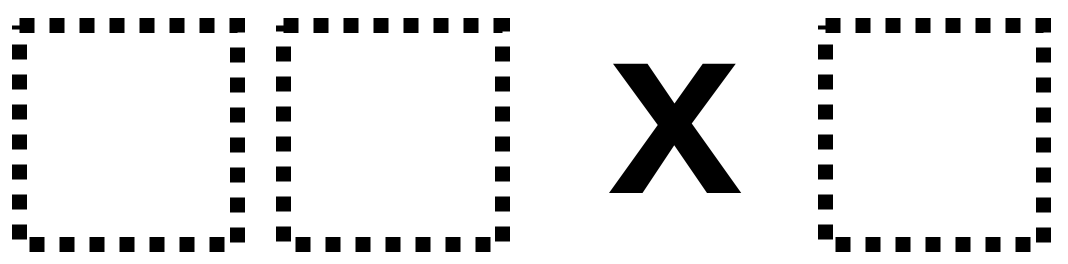# Multiplying a Two-Digit Number by a Single-Digit Number

Directions: Using the digits 1 to 4 at most one time each, fill in the boxes to make the largest possible product.### Hint

Where do you want to put your biggest number? Where do you want to put your smallest number? What would this look like as an array?

32 x 4 = 128 is the biggest product

Source: Robert Kaplinsky

## Simple Patterns 2

Directions: Using the digits 1 to 9 at most one time each, place a digit …

1.Love the format of the boxes above but do not see them on the worksheet.
Is it possible to have those boxes added to the worksheet?
Many thanks for your great website
sincerely
Robin Schwartz

•Hi Robin. The Open Middle Worksheet is not problem specific. So, for every problem students will need to add the boxes to their worksheet. Sorry!

•2.42 X 3 =126

3.32 x 4 = 128

4.5.32 x 4 = 128

6.20 x 3=60

•Zero was not a choice of digits.

7.32 x 4 128

8.32×4=128